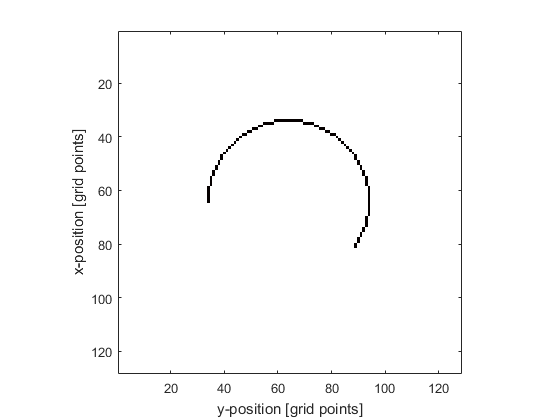# makeCircle

Create a binary map of a circle within a 2D grid.

## Syntax

```circle = makeCircle(Nx, Ny, cx, cy, radius)
circle = makeCircle(Nx, Ny, cx, cy, radius, arc_angle)
circle = makeCircle(Nx, Ny, cx, cy, radius, arc_angle, plot_circle)
```

## Description

`makeCircle` creates a binary map of a circle or arc (using the midpoint circle algorithm) within a two-dimensional grid (the circle position is denoted by 1's in the matrix with 0's elsewhere). A single grid point is taken as the circle centre thus the total diameter will always be an odd number of grid points. The plot that is displayed after calling `makeCircle(128, 128, 64, 64, 30, (215/180)*pi, true);` is given below.## Inputs

 `Nx, Ny` size of the 2D grid [grid points] `cx, cy` centre of the circle [grid points], if set to 0, the centre of the grid is used `radius` circle radius [grid points]

## Optional Inputs

 `arc_angle` arc angle for incomplete circle [radians] (default = `2*pi`) `plot_circle` Boolean controlling whether the circle is plotted using `imagesc` (default = false)

## Outputs

 `circle` 2D binary map of a circle

## Examples

`makeCartCircle`, `makeDisc`